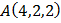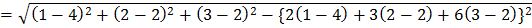# The distance of the pointfrom the line which passes through the pointand parallel to the vectoris a)b)c)d) 1

## Question ID - 54442 :- The distance of the pointfrom the line which passes through the pointand parallel to the vectoris a)b)c)d) 1

3537

(a)

The equation of line which passes through the pointand parallel to the vectorisDistance of pointfrom the lineNext Question :
 The angle between the linesandis a)b)c)d) None of these